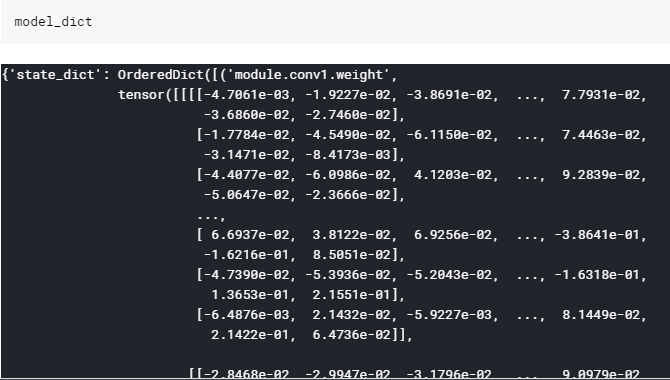# Using pretrained models weights

I’ve downloaded the weights of a resnet50 pretrained on places365 (http://places2.csail.mit.edu/models_places365/resnet50_places365.pth.tar)) and tried to load it, but i get this error:

. Any ideas of how to fix it?

Thanks

The problem is with your weight keys. fastai has different method of creating models, where we assign layers numbers sequentially.

To solve it you need to take your first model (the one pretrained on places365), and then modify it as below.

``````# For the CNN part
model = nn.Sequential(*list(model.children())

# want to use the default fastai head. I show the fastai default head
Flatten(),
nn.BatchNorm1d(4096),
nn.Dropout(0.25),
nn.Linear(4096, 512, bias=True),
nn.ReLU(inplace=True),
nn.BatchNorm1d(512),
nn.Dropout(0.5),
nn.Linear(512, num_outputs, bias=True)
)

``````

HI @kushaj, thanks a lot for taking the time to help me.

torch.load(‘path_weights’) returns a dictionary which hasn’t the attribute children. Do you have any ideas on how to proceed?

I also tried this:

But i get a similar error.

PS.:
Here’s how the dictionary returned by torch.load() looks like:I was using code from memory so messed up a bit. Here is a complete working example of the code.

``````import torchvision
model = torchvision.models.resnet18()

# Rename dict keys and remove head
model = nn.Sequential(*list(model.children()))
model = model[:-2]

Flatten(),
nn.BatchNorm1d(1024),
nn.Dropout(0.25),
nn.Linear(1024, 512, bias=True),
nn.ReLU(inplace=True),
nn.BatchNorm1d(512),
nn.Dropout(0.5),
nn.Linear(512, 10, bias=True)
)

# Combine the base model and custom head
# torch.save(model.state_dict(), 'model.pth')

learn = cnn_learner(data, models.resnet18, pretrained=False)

``````

When you run it in jupyter you will get the following message `IncompatibleKeys(missing_keys=[], unexpected_keys=[])`. Ignore it (just a bad way to say that torch.load worked properly).

1 Like

Hello and than ks again for taking the time to help me @kushaj, i really appreciate it.
I tried what you suggested and it still doesn’t work. Here’s a screenshot of the code followed by the error:

I also tried to load the weights before appending the head to the model, but get the same error

When you load the torchvision model, then load the new weights in it. After doing that, follow the other steps.

The reason you are getting the error is because when you do the abive steps the keys of your model are transformed to 0,1,2 while the resnet model that you want to load has the keys that you get inthe error.

The problem was that the key names were different.
I solved the problem by correcting their name.

Where did you find that fast ai replaces the head with this sequence @kushaj? I couldn’t find it

Flatten(),
nn.BatchNorm1d(1024),
nn.Dropout(0.25),
nn.Linear(1024, 512, bias=True),
nn.ReLU(inplace=True),
nn.BatchNorm1d(512),
nn.Dropout(0.5),
nn.Linear(512, 10, bias=True)
)

1 Like

Ignore it (just a bad way to say that torch.load worked properly).

Thanks–you saved me. That is really counterintuitive.